Aggregate Points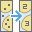The Aggregate Points tool uses area features to summarize a set of point features. The boundaries from the area feature are used to collect the points within each area and use them to calculate statistics. The resulting layer displays the count of points within each area using graduated symbols.

Workflow diagram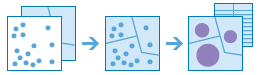Examples

Tip:

If your portal is configured to use Living Atlas content, you can use the state and county Living Atlas layers, which include population data, to aggregate the tornado data used in the workflow.

Usage notes

Aggregate Points is designed to collect and summarize point features within a set of boundaries. The input parameters must include points to be aggregated and aggregation areas.

You can provide the area layer to use for analysis, or you can generate bins of a specified size and shape (hexagon or square) into which to aggregate. The bin size specifies how large the bins are. If you are aggregating into hexagons, the size is the height of each hexagon and the width of the resulting hexagon will be 2 times the height divided by the square root of 3. If you are aggregating into squares, the bin size is the height of the square, which is equal to the width.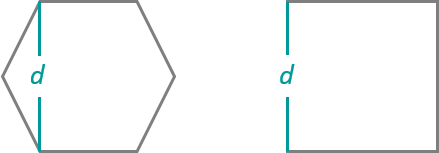Tip:

You can add a layer that is not in Map Viewer to the tool pane by selecting Choose Analysis Layer in the drop down menu.

The Keep areas with no points box is checked by default. When the box is checked, all of the areas used in the analysis will be included in the result, regardless of the location of points. Areas with no points will be empty and have a count of 0. When the box is unchecked, the areas with no points are completely removed from the result layer. Unchecking the box may have a significant effect on the result areas.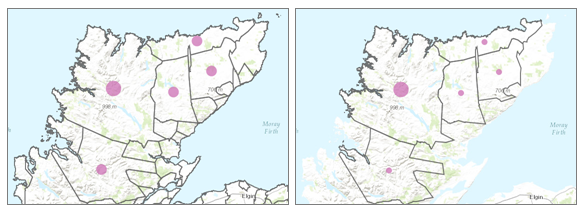The images show the difference in result layers when areas with no points are kept (left) or not kept (right).

The most basic aggregations will calculate a count of the number of points in each boundary. Basic statistics (sum, minimum, maximum, average, and standard deviation) can also be calculated on numerical fields. The statistics will be calculated on each area separately.

Statistical calculations can also be grouped using a field with categorical values. When statistics are grouped by a field, the statistics are calculated for both the area as a whole and for each group. Both statistics can be viewed in the result layer's pop-up. The overall statistics are given numerically and the grouped statistics are given in chart form within the pop-up. A summary table listing each feature and statistic by group field value will also be created. The Add minority, majority check box will return the groups with the lowest and highest counts and the Add percentages check box will return the percentage of points in the minority and majority groups.

If Use current map extent is checked, only the features in the input point layer and area layer visible within the current map extent will be analyzed. If unchecked, all features in both the input point layer and the area layer will be analyzed, even if they are outside the current map extent.

Limitations

Inputs must include a point layer and an area layer. Lines and areas cannot be aggregated within boundaries using the Aggregate Points tool.

How Aggregate Points works

Generating bins

Square and hexagonal bins can be generated for aggregation areas rather than aggregating points into an input area layer. The bins are generated in a custom, area-preserving projected coordinate system using the specified size dimensions to ensure the sizes are equal and appropriate for the area of interest. An appropriate equal-area projection and parameters are chosen based on the geographic extent of the input layers. Once bins are created, they are projected back to the coordinate system of the input data before being used in the analysis.

After the analysis is complete, the result is projected to Web Mercator for display (the default) or to the projection of your custom basemap. A Web Mercator projection may cause your results to appear distorted, especially for large bins or bins near the polar regions. These distortions are part of the display only and do not reflect an inaccurate analysis.

Equations

Standard deviation is calculated using the following equation: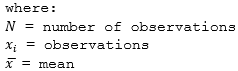Calculations

Point layers are summarized using only the point features within the input boundary. The results will be displayed using graduated symbols.

The figure and table below explain the statistical calculations of a point layer within a hypothetical boundary. The Population field was used to calculate the statistics (Sum, Minimum, Maximum, Average, and Std Deviation) for the layer.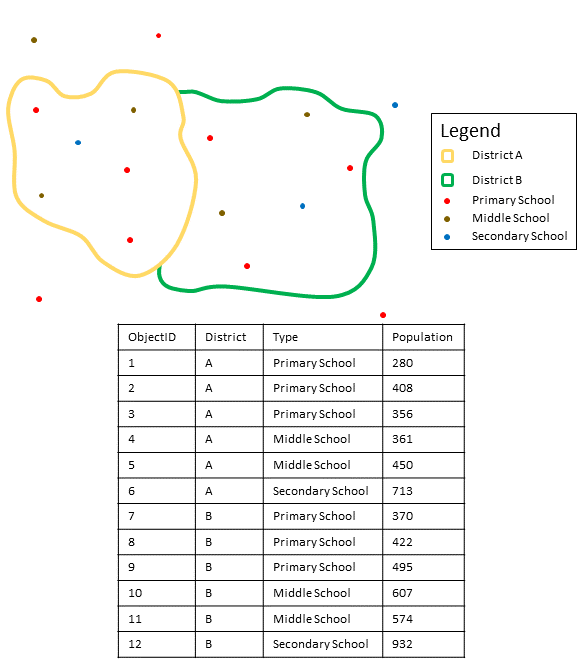Point layers are summarized using only points located within the boundary layer. An example attribute table is displayed above with values to be used in hypothetical statistic calculations.
StatisticResult District A

Sum

280 + 408 + 356 + 361 + 450 + 713 = 2,568

Minimum

Minimum of:

[280, 408, 356, 361, 450, 713] = 280

Maximum

Maximum of:

[280, 408, 356, 361, 450, 713] = 713

Average

2,568/6 = 428

Std Deviation= 150.79

A real-life scenario in which this analysis could be used is in determining the total number of students in each school district. Each point represents a school. The Type field gives the type of school (elementary, middle school, or secondary) and a student population field gives the number of students enrolled at each school. The calculations and results are given for District A in the table above. From the results, you can see that District A has 2,568 students. When running the Aggregate Points tool, the results would also be given for District B.

Similar tools

Use Aggregate Points to summarize points within areas. Other tools may be useful in solving similar but slightly different problems.

Map Viewer analysis tools

If you are trying to summarize lines or areas, use the Summarize Within tool.

ArcGIS Pro analysis tools

Aggregate Points performs the functions of the Spatial Join and Summary Statistics tools.

Aggregate Points is also available in ArcGIS Pro. To run the tool from ArcGIS Pro, your project's active portal must be running Portal for ArcGIS 10.5 or later. You must also sign in to the portal using an account that has privileges to perform standard feature analysis in the portal.